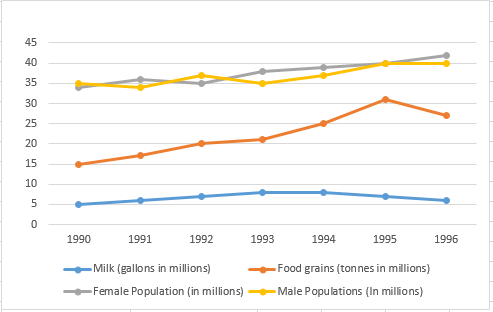### CAT 1997 Question 6

Instructions

The graph given below shows the quantity of milk and food grains consumed annually along with female and male population (in millions). Use the data to answer the questions that follow.Question 6

# When was the percapita consumption of milk the least?

Solution

The population is increasing steadily, but the milk consumed decreased significantly in 1996.
Hence, the only two years we need to check for the least per capita milk consumption are the first year (1990) and 1996.

Milk consumed in 1990 is 5 million gallons.
Total population in 1990 is 34+35 = 69 million.
Hence, per capita consumption of milk in 1990 is 0.072 gallon per person.

Milk consumed  in 1996 is 6 million gallons.
Total population in 1996 is 40+42 = 82 million.

Hence, per capita consumption  of milk in 1996 is 0.073 gallon per person.

Hence, the year with the least per capita consumption of milk is 1990.